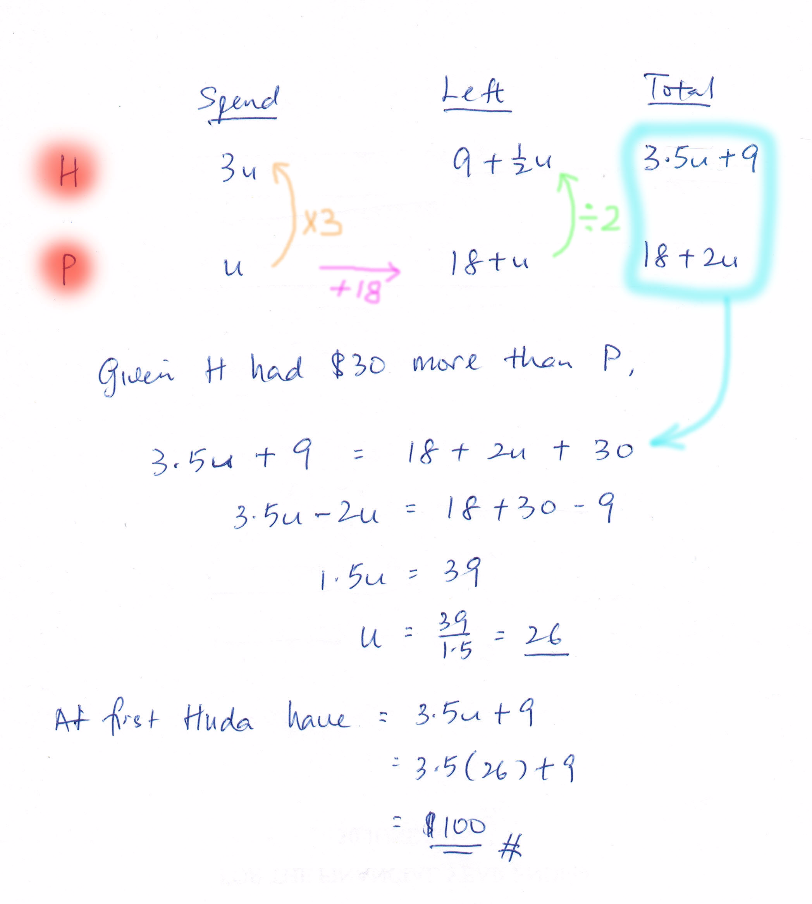# Question

Hi,

I really appreciate someone can help for this question. Thank you.

Huda and Peiqi went shopping with a sum of money. Huda had \$30 more than Peiqi. Huda spent thrice as much money as Peiqi. The amount Peiqi had left was \$18 more than what she had spent. Peiqi had twice as much money left as Huda. How much money did Huda have at first?

This is a Matrix question.

 Spend save Total Huda 6u 1u + 9 7u + 9 Peiqi 2u 2u + 18 4u + 18

7u + 9 = 4u + 18 + 30

7u – 4u = 48 – 9

3u = 39

1u = 13

7u = 7 × 13 = 91

91 + 9 = 100

0 Replies 2 Likes

Alternatively,

Huda :
money at first ——- 1u + 30
spending ——- 3p

Peiqi :
money at first ——- 1u
spending ——- 1p

1u – 1p = 1p + 18
1p ——- (1u – 18)/2
(1u + 30 – 3p) x 2 = 2u + 60 – 6p
2u + 60 – 6p = 1u – 1p
1u ——- 5p – 60 = 5 x [(1u – 18)/2] – 60
2u ——- 5u – 90 – 120
1u ——- (90 + 120)/(5 – 2) = 70
1u + 30 = 70 + 30 = 100

Ans : \$100.

0 Replies 1 Like0 Replies 3 Likes